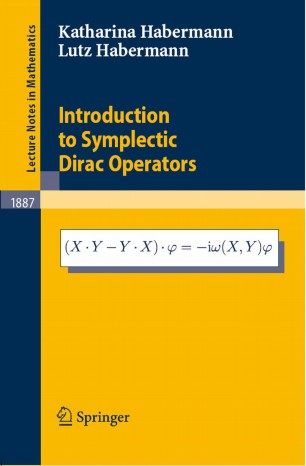# Introduction to Symplectic Dirac Operators

• Katharina Habermann
• Lutz HabermannBook

Part of the Lecture Notes in Mathematics book series (LNM, volume 1887)

1. Front Matter
Pages i-xii
2. Pages 1-19
3. Pages 21-34
4. Pages 35-48
5. Pages 49-66
6. Pages 67-79
7. Pages 81-96
8. Pages 97-100
9. Pages 101-113
10. Back Matter
Pages 115-124

### Introduction

One of the basic ideas in differential geometry is that the study of analytic properties of certain differential operators acting on sections of vector bundles yields geometric and topological properties of the underlying base manifold. Symplectic spinor fields are sections in an L^2-Hilbert space bundle over a symplectic manifold and symplectic Dirac operators, acting on symplectic spinor fields, are associated to the symplectic manifold in a very natural way. Hence they may be expected to give interesting applications in symplectic geometry and symplectic topology. These symplectic Dirac operators are called Dirac operators, since they are defined in an analogous way as the classical Riemannian Dirac operator known from Riemannian spin geometry. They are called symplectic because they are constructed by use of the symplectic setting of the underlying symplectic manifold. This volume is the first one that gives a systematic and self-contained introduction to the theory of symplectic Dirac operators and reflects the current state of the subject. At the same time, it is intended to establish the idea that symplectic spin geometry and symplectic Dirac operators may give valuable tools in symplectic geometry and symplectic topology, which have become important fields and very active areas of mathematical research.

### Keywords

Area Fourier transform Volume differential geometry manifold symplectic Dirac operators symplectic geometry symplectic manifold symplectic spinors

#### Authors and affiliations

• Katharina Habermann
• 1
• Lutz Habermann
• 2
1. 1.State and University Library GöttingenGöttingenGermany
2. 2.Department of MathematicsUniversity of HannoverHannoverGermany